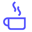a concise shot of dev knowledge
Become a Contributor
Concise shots of dev knowledge

RELATED TAGS

sort
algorithms

# How to implement Bubble sortEdpresso Team

The Bubble sort is a simple sorting algorithm that continues to swap adjacent values, if they are in the incorrect order, until all the values are sorted.

## The steps in the algorithm

To sort an array of size n in ascending order, it performs n-1 iterations and in each iteration:

1. It scans through the entire array.
2. It compares element at index i with the element at i+1.
3. If the element at ​position i is greater than the element at i+1, then the elements are swapped.

On average, this algorithm takes $O(n^2)$ time (where n is the size of the array). The algorithm can be optimized to take $O(n)$, if an array is already sorted in the required order, by breaking the outer for-loop if no element is swapped in the inner for-loop.

The following illustration shows the algorithm in action:

1 of 20

## Implementation

#include <iostream>
using namespace std;

int main() {
int arrayA[] = {3, 2, 1, 0};
// Finding the size of the array
auto n = end(arrayA) - begin(arrayA);
// Sorting array_A in ascending order
for(int it = 0; it < (n - 1); it++){
int flag = 0;
for(int i = 0; i < (n - 1); i++){
if(arrayA[i] > arrayA[i + 1]){
// Since the element at i is greater,
//swap elements at index i and i+1
int temp = arrayA[i];
arrayA[i] = arrayA[i + 1];
arrayA[i + 1] = temp;
flag = 1;
}
}
// Optimization. Break the outer for loop
// if no element is swapped in the inner for loop.
if(flag == 0){
break;
}
}
// Printing the sorted array
for(int i = 0; i < n; i++){
cout<< arrayA[i] << " ";
}
return 0;
}

RELATED TAGS

sort
algorithms
RELATED COURSES

View all Courses

Keep Exploring

Learn in-demand tech skills in half the time